# How to Find the Period of a Trig Function

Lesson Transcript
Instructor
Yuanxin (Amy) Yang Alcocer

Amy has a master's degree in secondary education and has been teaching math for over 9 years. Amy has worked with students at all levels from those with special needs to those that are gifted.

Expert Contributor
Kathryn Boddie

Kathryn has taught high school or university mathematics for over 10 years. She has a Ph.D. in Applied Mathematics from the University of Wisconsin-Milwaukee, an M.S. in Mathematics from Florida State University, and a B.S. in Mathematics from the University of Wisconsin-Madison.

In trigonometry, the period of a function refers to the distance of a function's wave. Learn how to find the period of a trig function by exploring the steps and working examples. Updated: 01/04/2022

## Steps for Finding the Period

Did you know that if you are given a trig function and asked to find the period, all you have to do is to look at one particular number and make a simple calculation?

That's right! Looking at this function, you might think you have to do something complicated, but you only need to worry about one of the numbers to figure out your period.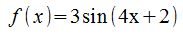The period is defined as the length of a function's cycle. Trig functions are cyclical, and when you graph them, you'll see the ups and downs of the graph and you'll see that these ups and downs keep repeating at regular intervals.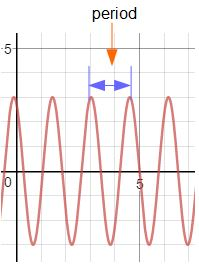All you have to do is to follow these steps.

#### Step 1: Rewrite your function in standard form if needed.

The first step you need to take is to make sure that your function is written in standard form: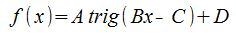The trig word in the function stands for the trig function you have, either sine, cosine, tangent, or cotangent. The A stands for the amplitude of the function, or how high the function gets. The B value is the one you use to calculate your period. When you divide your C by your B (C / B), you get your phase shift. The D stands for any vertical shift the function has. The vertical shift is how much above or below the x-axis the function is shifted.

The trig function from the beginning of this lesson, f(x) = 3 sin(4x + 2), already happens to be in standard form, so you don't have to do anything here.

#### Step 2: Label your A, B, C, and D values.

After rewriting your function in standard form if needed, now you can label your A, B, C, and D values.

For our example trig function, your A is 3, your B is 4, your C is -2, and your D is 0. Be careful here when it comes to labeling your C value. The C value is negative in the standard form, so if your C value is being added, then your C value is really negative.

#### Step 3: Calculate your period.

Your next step is to calculate your period using just the B value that you labeled in step two. You'll use two formulas to find your period.

If your trig function is either a sine or a cosine, you'll need to divide two pi by the absolute value of your B.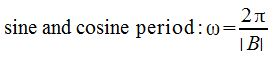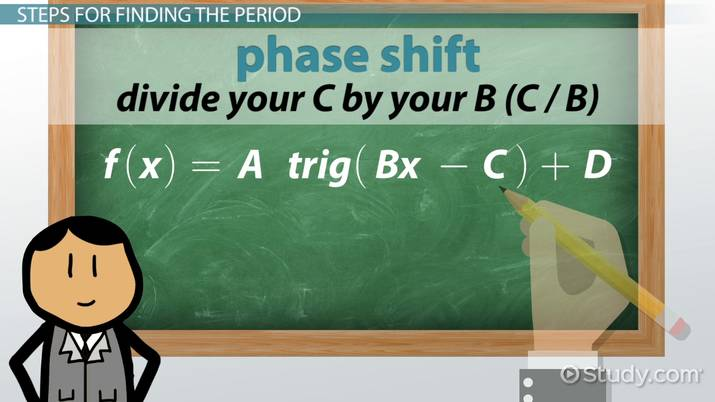An error occurred trying to load this video.

Try refreshing the page, or contact customer support.

Coming up next: How to Find the Phase Shift of a Trig Function

### You're on a roll. Keep up the good work!

Replay
Your next lesson will play in 10 seconds
• 0:00 Steps for Finding the Period
• 2:40 Solution
• 3:32 Example
• 3:58 Lesson Summary
Save Save

Want to watch this again later?

Timeline
Autoplay
Autoplay
Speed Speed

To unlock this lesson you must be a Study.com Member.

## Finding the Period of Secant and Cosecant

In the video lesson, we learned how to find the period of various trigonometric functions. If the trigonometric function is in standard form: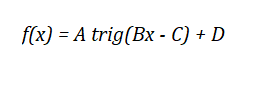where "trig" is sine, cosine, tangent or cotangent, we learned that the B value is used to find the period. The period for sine or cosine is given by: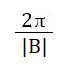And the period for tangent or cotangent is given by: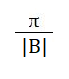But what if the trigonometric function is secant or cosecant?

## Definition and Graphs of Secant and Cosecant

The cosecant function is defined as one divided by sine and the secant function is defined as one divided by cosine.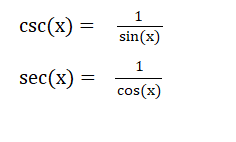The cosecant function has vertical asymptotes where the sine function is zero and the secant function has vertical asymptotes where the cosine function is zero.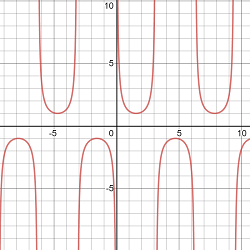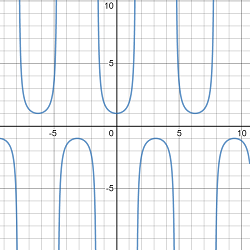Since secant and cosecant are related to sine and cosine, you may think that the period is calculated similarly to the period of sine and cosine - and you'd be right! If the secant or cosecant function is in standard form, the period of the function is given by:## Examples

1) Find the period of f(x) = 3sec(2x-5)+3

2) Find the period of g(x) = -2csc(3x+9) - 1

3) Find the period of h(x) = 5sec(-0.5x+1)-12

## Solutions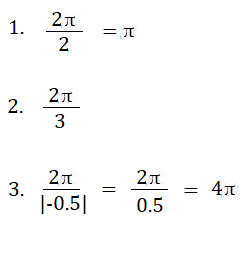### Register to view this lesson

Are you a student or a teacher?